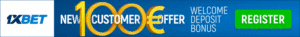# Number Divisibility Rules for 7, 13 and 17

A number (say N1) is divisible by another number (say N2) if the remainder is zero when N1 is divided by N2 (Example 18/9 = 2, with a remainder of 0, so 18 is divisible by 9). Have you ever found it difficult to check if a number is divisible by 7, 13 and 17? If yes, do not worry! Here are some easy rules to find out if a number is divisible by these numbers.

## Divisible by 7:

To check if a number is divisible by 7 just follow these simple steps:

1. Double the last digit of the original number and subtract it from the truncated number.
2. Apply step 1 over and over multiple times until you get a single digit or a double digit value.
3.  If the resultant value is divisible by 7, then the original number is divisible by 7.

Below are some of the examples that shows the application of this rule.

Example 1: 1234

1234 –> 123 – 8 = 115 –> 11 – 10 = 1.
1 is not divisible by 7. Hence, then number 1234 is not divisible by 7.

Example 2: 833

833 –> 83 – 6 = 77
77 is divisible by 7. Hence, the number 833 is divisible by 7.## Divisible by 13:

To check if a number is divisible by 13 just follow these simple steps:

1. Multiply four times the last digit of the original number and add it to the truncated number.
2. Apply step 1 over and over multiple times until you get a single digit or a double digit value.
3.  If the resultant value is divisible by 13, then the original number is divisible by 13.

Below are some of the examples that shows the application of this rule.

Example 1: 50674

50674 –> 5067 + 16 = 5083 –> 508 + 12 = 520 –> 52 + 0 = 52 –> 5 + 8 = 13
13 is divisible by 3. Hence, the number 50674 is divisible by 13.

Example 2: 4987

4987 –> 498 + 28 = 526 –> 52 + 24 = 76 –> 7 + 24 = 31 –> 3 + 4 = 7
7 is not divisible by 13. Hence, the number 4987 is not divisible by 13.

## Divisible by 17:

To check if a number is divisible by 17 just follow these simple steps:

1. Multiply five times the last digit of the original number and subtract it from the truncated number.
2. Apply step 1 over and over multiple times until you get a single digit or a double digit value.
3.  If the resultant value is divisible by 17, then the original number is divisible by 17.

Below are some of the examples that shows the application of this rule.

Example 1: 3961

3961 –> 396 – 5 = 391 –> 39 – 5 = 34
34 is divisible by 17. Hence, the number is divisible by 17.

Example 2: 45672

45672 –> 4567 – 10 = 4557 –> 455 – 35 = 420 –> 42 – 0 = 42
42 is not divisible by 17. Hence, 45672 is not divisible by 17.

Liked the article? Keep reading ReadScoops.com for more similar Education Scoops. Also, don’t forget to leave your comments on what you want to see up here next.## Read more:

About Phaneendra Varma
Fan of Cricket. Devotee of Sachin. Follower of the Cricket game since 2003 World Cup!